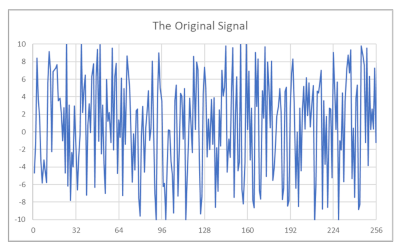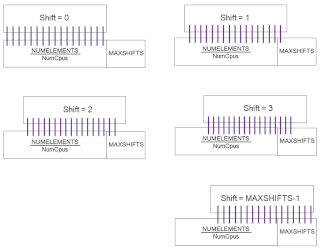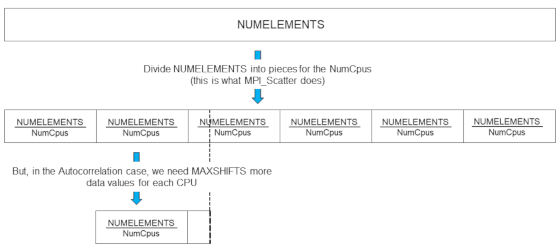## Project #7B

### Due: June 7 -- 23:59:59 -- No Bonus Days

This page was last updated: March 26, 2022

### Introduction

I like Wikipedia's definition of Autocorrelation:
"Autocorrelation, also known as serial correlation, is the correlation of a signal with a delayed copy of itself as a function of delay. Informally, it is the similarity between observations as a function of the time lag between them."

What does this actually mean? It means you take an apparently noisy signal, i.e., an array of length NUMELEMENTS random-looking values sampled over time:You then multiply each array element by itself and add them up:

```
Sums = A*A + A*A + ... + A[NUMELEMENTS-1]*A[NUMELEMENTS-1]
```
That will give you a large number because you are essentially taking the sum of the squares of the array elements. Big whoop. This is not interesting, so we ignore Sums. The other Sums[*], however, are much more interesting.

You then shift the array over one index and do the pairwise multiplication again. This gives a resulting value of

```
Sums = A*A + A*A + ... + A[NUMELEMENTS-1]*A
```

Now, if the signal is truly noisy, there will be positive and negative array values in there being multiplied together, giving both positive and negative products. Many of these will cancel each other out. So, by doing this shift before multiplying, you will get a much smaller sum.

You then shift over by 2 indices and do it again,

```
Sums = A*A + A*A + ... + A[NUMELEMENTS-2]*A + A[NUMELEMENTS-1]*A
```
and do it again, and do it again, and so on.

In the Sums and Sums lines of code above, notice the required wrap-around, back to A and A. Clearly, the logic to do this isn't hard, but it would help our flat-out parallelism if we didn't have to do that. More on this later.What does this do? You then graph these resulting Sums[*] as a function of how much you shifted them over each time. If there is a secret sine wave hidden in the signal, well, let's just say that you will notice it in the graph of the Sums[*]. It's not even subtle. It will slap you in the face.

The presence of that harmonic will make the Sums[*] array be all positives for a while, then all negatives, then all positives, etc.

Scientists and engineers use the autocorrelation technique to see if there are regular patterns in a thought-to-be-random signal. For example, you might be checking for 60-cycle hum contamination in a sensor signal, or intelligent life elsewhere in the universe, etc.

The problem is that these signals can be quite large. Your job in this project is to implement this using MPI, and compare the performances across different numbers of processors.

### Requirements:

1. Read one of the signal files, either in text format bigsignal.txt or in binary format bigsignal.bin. Each file has 8,388,608 (=8*1024*1024 =8M) signal ampliudes in it. You can look at the text version if you want.

2. To be sure that you downloaded the complete file, check the file size against:
 bigsignal.bin 33554432 bigsignal.txt 75497472

To read the signal data, see the code below.

3. A not-paralleled C/C++ way of doing the multiplying and summing would be:
```
for( int s = 0; s < MAXSHIFTS; s++ )
{
float sum = 0.;
for( int i = 0; i < NUMELEMENTS; i++ )
{
sum += A[i] * A[i + s];
}
Sums[s] = sum;
}
```

Note that this works because A was dimensioned twice the size as the number of signal values, and then two copies of the signal were placed in it. This allows you to do the autocorrelation in one set of multiplies instead of wrapping around. So, your program will really be doing:

```
Sums = A*A + A*A + ... + A[NUMELEMENTS-2]*A[NUMELEMENTS-2] + A[NUMELEMENTS-1]*A[NUMELEMENTS-1]
Sums = A*A + A*A + ... + A[NUMELEMENTS-2]*A[NUMELEMENTS-1] + A[NUMELEMENTS-1]*A[NUMELEMENTS]
Sums = A*A + A*A + ... + A[NUMELEMENTS-2]*A[NUMELEMENTS]   + A[NUMELEMENTS-1]*A[NUMELEMENTS+1]
. . .
```

We won't do all NUMELEMENTS Sums[ ], we will just do MAXSHIFTS of them. That will be enough to uncover the secret sine wave.

4. Do this using MPI parallelism.
Each processor will be responsible for autocorrelating (NUMELEMENTS/NumCpus) elements.
(However, it will need (NUMELEMENTS/NumCpus)+MAXSHIFTS elements to do it.)5. Scatterplot the autocorrelation Sums[*] vs. the shift. Even though there will be MAXSHIFTS Sums[*] values, only scatterplot Sums ... Sums.

There is a secret sine-wave in the signal. Scatterplotting Sums ... Sums will be enough to reveal it. Don't worry -- if you have done everything correctly, it will be really obvious.

Don't include Sums in the scatterplot. Sums will be a very large number and will cause your auto-scaled vertical axis to have larger values on it than is good to view the secret sine-wave pattern.

6. Tell me what you think the secret sine wave's period is, i.e., what change in shift gives you a complete sine wave?

7. Draw a graph showing the performance versus the number of MPI processors you use. Pick appropriate units. Make "faster" go up.

8. Turn into Teach:
1. All your source code files (.c, .cpp).
2. Your commentary in a PDF file.

9. Your commentary PDF should include:
1. Show the Sums{1] ... Sums vs. shift scatterplot.
2. State what the secret sine-wave period is, i.e., what change in shift gets you one complete sine wave?
3. Show your graph of Performance vs. Number of Processors used.
4. What patterns are you seeing in the performance graph?
5. Why do you think the performances work this way?

### Skeleton Code:

```
#include <stdio.h>
#include <math.h>
#include <mpi.h>

// which node is in charge?

#define BOSS 0

// files to read and write:

#define BIGSIGNALFILEBIN	(char*)"bigsignal.bin"
#define BIGSIGNALFILEASCII	(char*)"bigsignal.txt"
#define CSVPLOTFILE		(char*)"plot.csv"

// tag to "scatter":

#define TAG_SCATTER		'S'

// tag to "gather":

#define TAG_GATHER		'G'

// how many elements are in the big signal:

#define NUMELEMENTS	(8*1024*1024)

// only do this many shifts, not all NUMELEMENTS of them (this is enough to uncover the secret sine wave):

#define MAXSHIFTS	1024

// how many autocorrelation sums to plot:

#define MAXPLOT		 256		// an excel limit

// pick which file type to read, BINARY or ASCII (BINARY is much faster to read):
// (pick one, not both)

#define BINARY
//#define ASCII

// print debugging messages?

#define DEBUG		true

// globals:

float * BigSums;		// the overall MAXSHIFTS autocorrelation array
float *	BigSignal;		// the overall NUMELEMENTS-big signal data
int	NumCpus;		// total # of cpus involved
float * PPSums;			// per-processor autocorrelation sums
float *	PPSignal;		// per-processor local array to hold the sub-signal
int	PPSize;			// per-processor local array size

// function prototype:

void	DoOneLocalAutocorrelation( int );

int
main( int argc, char *argv[ ] )
{
MPI_Status status;

MPI_Init( &argc, &argv );

MPI_Comm_size( MPI_COMM_WORLD, &NumCpus );
int  me;		// which one I am
MPI_Comm_rank( MPI_COMM_WORLD, &me );

// decide how much data to send to each processor:

PPSize    = NUMELEMENTS / NumCpus;		// assuming it comes out evenly

// local arrays:

PPSignal  = new float [PPSize+MAXSHIFTS];	// per-processor local signal
PPSums    = new float [MAXSHIFTS];		// per-processor local sums of the products

// read the BigSignal array:

if( me == BOSS )	// this is the big-data-owner
{
BigSignal = new float [NUMELEMENTS+MAXSHIFTS];		// so we can duplicate part of the array

#ifdef ASCII
FILE *fp = fopen( BIGSIGNALFILEASCII, "r" );
if( fp == NULL )
{
fprintf( stderr, "Cannot open data file '%s'\n", BIGSIGNALFILEASCII );
return -1;
}

for( int i = 0; i < NUMELEMENTS; i++ )
{
float f;
fscanf( fp, "%f", &f );
BigSignal[i] = f;
}
#endif

#ifdef BINARY
FILE *fp = fopen( BIGSIGNALFILEBIN, "rb" );
if( fp == NULL )
{
fprintf( stderr, "Cannot open data file '%s'\n", BIGSIGNALFILEBIN );
return -1;
}

fread( BigSignal, sizeof(float), NUMELEMENTS, fp );
#endif

// duplicate part of the array:

for( int i = 0; i < MAXSHIFTS; i++ )
{
BigSignal[NUMELEMENTS+i] = BigSignal[i];
}
}

// create the array to hold all the sums:

if( me == BOSS )
{
BigSums = new float [MAXSHIFTS];	// where all the sums will go
}

// start the timer:

double time0 = MPI_Wtime( );

// have the BOSS send to itself (really not a "send", just a copy):

if( me == BOSS )
{
for( int i = 0; i < PPSize+MAXSHIFTS; i++ )
{
PPSignal[i] = BigSignal[ BOSS*PPSize + i ];
}
}

// have the BOSS send to everyone else:

if( me == BOSS )
{
for( int dst = 0; dst < NumCpus; dst++ )
{
if( dst == BOSS )
continue;

MPI_Send( &BigSignal[dst*PPSize], ???, ???, ???, ???, ??? );
}
}
else
{
MPI_Recv( PPSignal, ???, ???, ???, ???, ???, &status );
}

// each processor does its own autocorrelation:

DoOneLocalAutocorrelation( me );

// each processor sends its sums back to the BOSS:

if( me == BOSS )
{
for( int s = 0; s < MAXSHIFTS; s++ )
{
BigSums[s] = PPSums[s];		// start the overall sums with the BOSS's sums
}
}
else
{
MPI_Send( PPSums, ???, ???, ???, ???, ??? );
}

// receive the sums and add them into the overall sums:

if( me == BOSS )
{
float tmpSums[MAXSHIFTS];
for( int src = 0; src < NumCpus; src++ )
{
if( src == BOSS )
continue;

MPI_Recv( tmpSums, ???, ???, ???, ???, ???, ??? );
for( int s = 0; s < MAXSHIFTS; s++ )
BigSums[s] += tmpSums[s];
}
}

// stop the timer:

double time1 = MPI_Wtime( );

// print the performance:

if( me == BOSS )
{
double seconds = time1 - time0;
double performance = (double)MAXSHIFTS*(double)NUMELEMENTS/seconds/1000000.;	// mega-elements computed per second
fprintf( stderr, "%3d processors, %10d elements, %9.2lf mega-autocorrelations computed per second\n",
NumCpus, NUMELEMENTS, performance );
}

// write the file to be plotted to look for the secret sine wave:

if( me == BOSS )
{
FILE *fp = fopen( CSVPLOTFILE, "w" );
if( fp == NULL )
{
fprintf( stderr, "Cannot write to plot file '%s'\n", CSVPLOTFILE );
}
else
{
for( int s = 1; s < MAXPLOT; s++ )		// BigSums is huge -- don't use it
{
fprintf( fp, "%6d , %10.2f\n", s, BigSums[s] );
}
fclose( fp );
}
}

// all done:

MPI_Finalize( );
return 0;
}

// read from the per-processor signal array, write into the local sums array:

void
DoOneLocalAutocorrelation( int me )
{
MPI_Status status;

if( DEBUG )	fprintf( stderr, "Node %3d entered DoOneLocalAutocorrelation( )\n", me );

for( int s = 0; s < MAXSHIFTS; s++ )
{
float sum = 0.;
for( int i = 0; i < PPSize; i++ )
{
sum += PPSignal[i] * PPSignal[i+s];
}
PPSums[s] = sum;
}
}
```